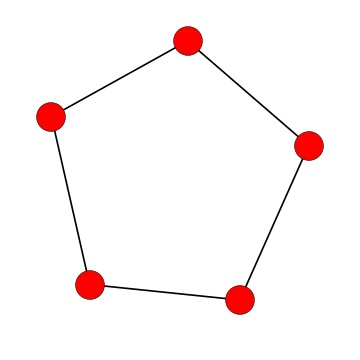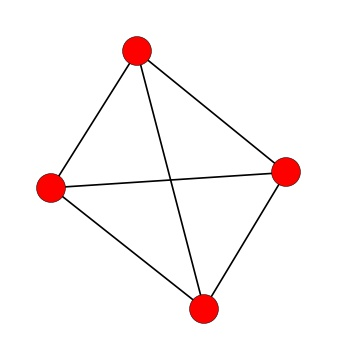Probability

# Planar GraphsIs this graph planar?Is this graph planar?

Suppose a graph $$G$$ is not planar. If we add an edge to $$G$$ to connect two previously disconnected nodes to create the graph $$G'$$, is $$G'$$ planar?

Suppose that there are three houses $$A, B, C$$ and three utilities 1, 2, and 3 each of which needs to be connected by a wire to all three houses. Assuming the utilities and the houses are all points (nodes), is there a way to position them and the wires (edges) such that no two wires overlap?

Note: this is the same as asking whether $$K_{3,3}$$ is a planar graph.

Suppose $$G$$ is a planar graph. If we create $$G'$$ by adding a single edge to $$G$$, is $$G'$$ planar?

×